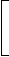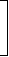# Aptitude - True Discount - Discussion

### Discussion :: True Discount - General Questions (Q.No.4)

4.

A man wants to sell his scooter. There are two offers, one at Rs. 12,000 cash and the other a credit of Rs. 12,880 to be paid after 8 months, money being at 18% per annum. Which is the better offer?

 [A]. Rs. 12,000 in cash [B]. s. 12,880 at credit [C]. Both are equally good

Explanation:

P.W. of Rs. 12,880 due 8 months hence
= Rs.12880 x 100100 +18 x 812
 = Rs.12880 x 100112
= Rs. 11500.

 Tanu said: (May 19, 2011) Why that 100 is adding in denominator? Can any one plz explain properly......

 Omer Bin Mohd(Hyd)9959542712 said: (Jul 13, 2011) Hi Tanu As per formula of Present Worth (P.W) 100 x Amount/100 + (R = Rate perannum x T = Time Year) 12000 is better as such 12880 is due amount after 8 Months =100+18*8/12 = 100+12 =112

 Rad said: (Aug 1, 2011) Can any one please explain properly ?

 Bj said: (Aug 6, 2011) Not understandable. Explain Formula clearly.

 Sanjay said: (Sep 14, 2011) Hey let me explain here given P.W. Is 12000 now if we calculate the P.W. Of Rs. 12880 which will recieved after 8 months thus formula P.W.=100*amt./100+(R*T) Is used.

 Madhu.T said: (Sep 27, 2011) Just see my ans:- By taking after 8 months how much we will get excess money = 12880-12000 = 880/- ..........(Pls remind this), If we take cash 12000/- now it self the interest would be after 8 months @ 18% P.A = 12000*(18/100)*(8/12) = 1440 so instead of going for credit, cash is better.

 Anonyma said: (May 28, 2012) After 8 does not mean in 4 months as the previous question. The question does not say that in 8 months but after 8months starting from 4 left months of the year the man will start to pay right?

 Deepak said: (Jun 2, 2012) Hey Guys... I am little worried about this solution. Let me put my thoughts below. Cash = 12000 Today Interest rate 18% /Annum. After 8 Months Interest will be 12000*(8*18%/12)= 1440. If I will not pay 12000 now , then my worth will be 12000+1440 = 13440. If I pay 12800 after 8 months, still I will have 13440-12880= 560 in my hand. If I pay 12000 now, then I cant have 560 in my hand which is a interest from this cash. So I feel 12880 after 8 months is best answer.

 Amit said: (Aug 23, 2012) It should be mentioned "better from which side"...

 Akshay said: (Jun 17, 2013) Agreed with @Deepak. Simply. I would like to pay 11500(PW) instead of paying 12000(PW).

 Jasjit said: (Jul 21, 2013) @Deepak. We are only concerned about the two cases so option A is better. You are considering 18% rate without taking the dealer's scheme so its impossible.

 Prasanna \$Araf said: (Aug 2, 2014) I agree with deepak as the solution says if I pay 12800 later thats just 11500 now so we are saving 500 here. So it's better to pay later.

 Ssr said: (Aug 5, 2014) The question here asks which option is better for owner of the scooter. So by explanation of Madhu.T cash is better than credit. Because if we take cash now and give it for interest we get 1440 after 8 months at 18% interest per annum. But if we accept credit we only get 880 after 8 months.

 Sudheer said: (Apr 17, 2015) What is P.W? Anyone can explain?

 Nivrutti said: (Oct 8, 2015) Why are you taken 100 please tell me anyone?

 Owmker Das said: (Nov 15, 2015) Present Value of 12880 is 11500. This option costs lower than present 12000. So, answer should be 'on credit'. Kindly explain anyone?

 Vishal said: (Dec 28, 2015) As I see it we only need to compare the two either pay cash or you pay 12880 which includes your 8 months interest @18%. We don't have the option of receiving 12000 after 8 months.

 Kavya said: (Jun 5, 2016) Amount= P.W + I. I = (P.W * T * R)/100. So, A = P.W+ (P.W * T * R)/100. 100(A) =100 * P.W + P.W * T * R. P.W = 100A/100 + T * R. A = 12880 (A is the total amount obtained after 8 months). 12000 -> obtained P.W.

 Raja said: (Sep 12, 2016) Thank you @Madhu for the best explanation you gave. @Deepak, this deal is for a dealer not for the customer. For the customer, the best one is of 12880. Otherwise, he'll charge with an imaginary amount of 13440. And for the dealer, the best one is of 12000 in cash.

 Rajesh Saha said: (Feb 7, 2017) Well explained, I agree @Deepak.

 Prathamkumar said: (Feb 25, 2017) But how the answer is A? I am not getting it.

 Seema said: (Jun 30, 2017) Well said, I agree @Raja.

 Khandaker Sajib Ahmed said: (Aug 1, 2017) P = A/(1 + nr). Where: P = The present value of the amount to be paid in the future, A = The amount to be paid, r = The interest rate, n = The number of years from now when the payment is due.

 Umang said: (Sep 1, 2017) Since the man sells the better offer is 12000 (Profit as a seller). If the man buys the better offer is 12800 (Profit as a buyer).

 Naman Jain said: (Sep 20, 2017) Not understood the soluation can you elaborate it please?

 Shahin said: (Oct 9, 2017) In numerator why 100 is multiplied with amount?

 Vikas said: (Jan 12, 2018) The question is which one is a better option for the seller not for the buyer, so if he sells it for cash of Rs. 12000 but if he sells for credit he will only receive only Rs. 11500 (solved using formula). Hence better option is to sell it for cash.

 Mohit Sharma said: (Apr 26, 2018) Pv = Fv/(1+n*r) simple interest, Pv = 12880/(1+.18/12*8), = 12880/1.12, PV = 11500.

 Yash said: (Aug 18, 2018) Agreed @Vikas.

 Logeshwari said: (Sep 11, 2019) Thanks all for explaining it.

 Sonu said: (Aug 26, 2020) Thanks for explaining @Kavya.

 Gitanjali Kumari said: (Oct 14, 2020) Thanks @Madhu.

 Abirami D said: (Nov 20, 2020) Thanks for deriving the formula @Kavya.